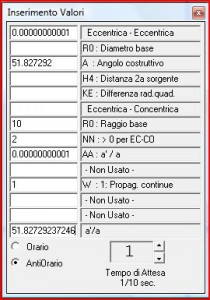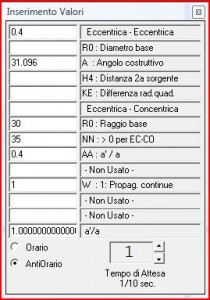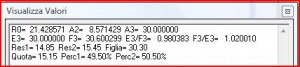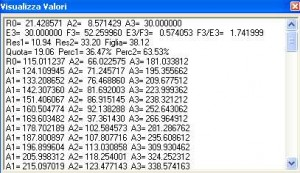# Chap.3.6 A)The “undulatory composition”…

## Chapter 3.6  The “undulatory composition”: from  photonic waves  to galactic waves. Part Two).

§ 12. In the article Gravity and the other “forces” (cap.4 c)) the figure having   number 12 is the number 4 and it  represents the  ” a rosette”  orbiting of  two equal masses. We have reproduced it here to show in this case the geometry of  the orbiting of a pair of photons, during which the undulatory composition of the  figg.10, 11, 13, 14, is periodically repeated.

However, in nature, the design can multiply its configuration for a multiple number of photonic pairs mutually and orderly orbiting, diametrically disposed, whose  undulatory result is the confluence  in a same composed wave not of a single pair of waves, but of more pairs. In this case, those ones pour  in the composed wave, as in a common reservoir,  their gravitational intensity:  D3, which is the sum of the intensities converged in the composed wave, will be 20 * n pairs of photons and the resulting distribution will see the original wave of the daughter  propagation enriched many times the conventional value 10 as  the competing pairs are.

Naturally the parent photonic  propagations emerge from the composition halved in their  intensity: this does not mean that we will now have concentric propagations of intensity  not equal to 10, as in the original framework, but 10 * n with n> 1.

§ 13. Fig.16 is reproduced in the first work by the owner of these pages (Unigravitational Field Physics, Naples 1969): it belongs, therefore, to the archeology of the new physics, and as such,  it is devoid of mathematical precision possessed by today computational tools and design. However, besides being dear in the memory  of the efforts of those passed and hard times, it is very suitable to illustrate the present discussion, showing the non-contingent value of its original processing.

It shows six photonic centers from  AI  to AVI , each with one wave in emission and the dashed trend – clockwise or counterclockwise – of the eccentric propagation, that goes to channel (we repeat, in a graphically imprecise way)  in a common composed wave (in  equatorial projection,   the central circumference), from which a concentric daughter propagation comes out.

On that one,  the points of intensity undouble  and diverge along six  controversial pairs of spirals of Archimedes, because the photonic  pairs,  diametrically arranged,  have antiparallel axis so they give to those points a rotatory motion of  opposite direction.

A seventh photonic center, AVII, is represented as punctiform,  in  circular orbiting around the nucleus of the first six, meaning that its eccentric propagation, not drawn, is directed  not in the same composed wave  of the other six, but into an already propagated  wave, the one markedly  dotted. The result of this additional composition, which occurs outside the geometrical locus of the composition among the first six photons, is  not represented.

§ 14. The central composed wave, which includes the gravitational intensity of the six photons, will have an intensity  equal to  20 * 3 pairs, but the concentric daughter wave comes out with an intensity equal to 10 * 3 (§ 12), the global value that can be stored for each wave during the next propagation (while the punctual intensity is decreasing). The spirals of Archimedes – six clockwise  and six counterclockwise –  constitute as many magnetic “force lines” or magneto-gravitational, or more precisely ” lines of  gravitational intensification.”

In cap.VI (The gravitational structuring) we will see how these lines of force, dragged to spiral by the mutual orbiting of photons (as  of any particle or body),  also draw, for  the phenomenon of “pulsation” and “interincidence”, geometric loci, relatively fixed in space, of gravitational densification of the external matter, which give rise to strict natural forms, ie to “structures” or “bodies”.

The absolute ignorance of the official physics about the phenomena of the gravitational structuring prevents an understanding of the causes that – for example – join, not because of mere “similarities” and “analogies”, but in an etio-genetic way, ie for basic laws, the draw of  fig.16 with an  hexapetal flower (fig.17)…

…or with the diffractogram of a crystal of beryllium (fig.18).

Simply replace the reciprocal positions of the photons with those of much larger particles such as atoms:  the alignments of those ones at the  intersection of the lines of force form edges and vertices of a crystal, and those  lines of force and those undulating contours  determine petals and calyx of a flower.

§ 15. Let us examine the composition between concentric propagation born from six photons (equintense sources) and the elementary eccentric one (not drawn) of a seventh photon: composition that takes place in a different place in space, namely the one of the spherical wave marked by strong dash  in fig.16. From such a composed wave a  new daughter propagation  has to  come out, that is also not drawn, eccentric in this case, because the two sources – the group of six photons and single photon – are disequintense.

Let’s see the result of it, at first only mathematically, then graphically too. Then we take the formulas of § 9 and we set E3 as intensity of the  single photon, F3 as that of  the daughter  propagation (concentric) of the three photonic pairs. We will have:

E3 = 10F3 = 30D3 (composed wave, marked dash) = 40

Res1 = 100 / 40 = 2,5

Res2 = 900 / 40 = 22,5

Figlia = 2 * 300 / 40 = 15

Quota = 300 / 40 = 7,5

D3 = Res1 + Res2 + Figlia = 40

E3 = Quota + Res1 = 10

F3 = Quota + Res2 = 30

§ 16. There will be now, therefore,  in the universe,  two propagations of intensity higher than the basic photonic one, which is equal to 10, and namely Res2 (concentric) = 22.5 and Figlia (eccentric) = 15.

More eccentric propagations of value 15, in turn, will converge in pairs in further composed waves of value 30 * n pairs, from which daughters concentric propagations will come out of value n * 15 pairs, in addition to those previously considered of value 10 *n.

It is, at this point, quite clear that the process will continue indefinitely, by increasing, without limit, sizes and intensity of gravitational waves, until – as it was said – those that convey the galaxies.

§ 17. It  is extraordinarily interesting to note that the formulas of  gravitational distribution, that we discovered, mean mathematically that in the GRAVITATIONAL CONJUGATION , ie in the birth of a daughter propagation following the undulatory composition, the more intense propagations are constantly advantaged compared to the  less intense ones: these  last  are depleted as a percentage more than the others, because they  contribute to the conjugation with a share exactly equal to that of the strongest competitors. The disadvantage is  greater as  greater is the disproportion between the more intense source and the less intense one, which gives itself completely, to an infinite limit,  as a contributive share to the daughter  propagation.

We see, in fact, from the formulas of § 9 that, if we tend E3 to infinity compared to the value of F3, it follows that D3 tends to E3, Res1 tends to E3, Res2 tends to zero, Figlia tends to 2 * F3, Quota tends to F3. This means that the infinitely more intense source, helping its daughter with a share F3 tending for it to zero, does not miss anything of its intensity; the weak source on the contrary provides a share equal to  itself, namely it disappears.

This is extremely important for the economy of the universe, as it prevents the fragmentation and the dispersion of the gravitational force among many sources having a  too weak intensity.

§ 18. We pass now to the graphical aspect of the problem posed by the fig.16. The convention used in the program (§ 9), of adjusting the diameters of the primary waves of the two parent  propagations  to the values ​​of their intensity, now forces to a choice between two graphic-mathematical solutions .

The first approaches graphically to the design of fig.16, but it does not correspond to the mathematical data of the problem. In the second case  the reverse occurs. We propose  both  (figg.19 and 20) for their didactic effectiveness, so to speak.
In fig.19 a) the photonic propagation (not present in fig.16) consists of  the propagated  wave of  a concentric one, as marked in fig.16, with radius √2 compared to the one of the original wave (internal):

In fig.19 b) a new vertical axis intersects the barycentre of the daughter  propagation, of which a propagated  wave appears, which rotates counterclockwise around the barycentre:

The barycentre of the daughter  propagation divides, according to the cosmological equation, the diameter of the wave consisting  of two parts inversely proportional to the intensity of the two sources.

Be very careful: in the cosmological equation, D – defined as the distance between two gravitational sources – is not geometrically the distance between the centers of mass of two bodies (or between the barycentres of  the respective propagations), but it is the measure of the diameter of the wave of composition between the two bodies. So in the figure, if the barycentre of the concentric  can be thought of as the center of mass of a body  and the barycentre of the photonic  propagation as the center of the photon, D is not joining them, but the entire diameter of the composed wave among  ​​ their propagations, on which  the equation calculates the above division into two parts through the barycentre of the daughter propagation. In other words, it is as if the two sources were located at the  ends of that diameter.

The fig.19 c) shows the development of the three propagations: the residual parents in  gray – eccentric and concentric photonics – and eccentric daughter. The program assumes a concentric one  in which the concurrent propagations are antiparallel in pairs, and therefore there is perfect balance in the daughter between clockwise rotating impulse and counterclockwise impulse: it follows that the eccentric propagation  conveying itself additionally counterclockwise, gives priority to this sense in the daughter propagation. Obviously, in nature this is not always true, because there are concentrics with a prevalence of one of the two senses: in this case the result will be different from that conventionally assumed.

The fig.19 in its three stages is carried out with the subprogram COMPOSIZIONE, by inserting the following inputs:1. ECCENTRICA-CONCENTRICA  (EC abbreviation): R0 = 10;  NN = 2, AA = 0.00000000001, W = 1.              At the top (ECCENTRICA-ECCENTRICA, EE) in the first rectangle  enter the same value of AA (ie a ‘/ a), which will give in last rectangle the value of A (constructive angle) correspondent to the first and due to that abbreviation: in this case, approximate, 51.827292. ASSI CARTESIANI.
2. Type AVANTI to a drawing in steps;  PLAY for  fig. 19 c).

§ 19. If, after building the fig.19, we go to find the mathematical data ​​in the VISUALIZZA VALORI window (fig. 19 d)), we will not find those of our problem: namely, the composition between a photon and the system of the other six (which we already can define “particle”). In fact, the program has calculated  the intensity of F3 (concentric) on the relationship between the value 10, diameter of the photonic  primitive wave, and that of  the concentric primitive wave, and it has obtained a value much higher than 30 of the propagation of the three photonic pairs (§ 15): F3 = 203.728279, which is higher than 20 times to E3, instead of being in a relation of 3 to 1. All other mathematical values ​​are related to this situation and not to the original one.

The graphically visible difference of the above is in the position of the barycentre of the daughter propagation, for which the diameter of the composed wave is divided according to the percentages 4.68 and 95.32 instead of 25 and 75, as it would be  in our case.
But however – as noted above – we can use mathematics to solve exactly the problem, apart from the  graphical similarity with the fig.16. To respect the convention wanted by the program and in the same time the actual mathematical values ​​of our case, we calculated that the composition must be carried out with a concentric wave much more advanced than that represented in fig.16, that is, with a radius √92. The result is, with sufficient approximation, fig. 20 (which is realized by changing only, between the inputs, NN = 92):

The values ​​found now in the window are those already calculated in § 15:

E3 = 10; F3 = 30.038096

Res1 = 2.50; Res2 = 22.54; Figlia = 15

Quota = 7.50; Perc1 = 24.98; Perc2 = 75.02

The barycentre now divides the diameter according to the wanted percentages and  around it the eccentric daughter propagation,  geometrically correct, is developed: you can clearly see the undulatory counterclockwise stripe  of densification, formed by the  eccentric waves outside the central design.

§ 20. Our reasoning proceeds according to an exhaustive  approach, that is  to consider all the possibilities of the occurrence of a phenomenon – in this case, the undulatory composition between  two sources from the photonic ones – as well as all roads leading  to demonstrate in a complete way a theorem.

We have seen that there is no limit to the increase in intensity and size of the waves according to the law of composition, and that from Figs. 19 and 20 two different eccentric daughter propagations have come out:  the first one  of intensity 19.06 and with a relation a ‘/ a = E3 / F3 = 0.049;  the second one of  intensity 15 and with a’ / a = 0.33. They are  composed  propagations. All that remains is to show the readers how to manage the subprogram COMPOSIZIONE at their own discretion, to verify the various cases of undulatory  composition among  sources of any entity and mutual relation a ‘/ a  and  to study the graphical  and mathematical results.

§ 21. We specify the geometric condition necessary because the composition can take place. There are two possibilities:

a) The two parent propagations have a common equatorial plan.

b) They have two different equatorial plans, but intersecting along the line joining the barycentres of the same propagations. In this second case, the daughter propagation develops along its equatorial plan, which intersects the other two along the same joining and it  is angled with respect to them with  angles inversely proportional to the intensity of the two parent sources: ie, according to the same law that establishes the proportion between the two sides of the diameter of the composed wave.

The composed gravitational propagation is progressively always  the result between just  two sources, except in the case of multiple sources, which contribute to the same concentric propagation, as seen above.

We confirm  what has been repeatedly stressed, namely that it is possible the composition between  eccentric propagations or between concentric and eccentric ones, but not  between concentric ones only.

§ 22. It has been said in § 5 that the gravitational spiral is determined by a constructive angle, that is the acute angle which is the basis of a right triangle inscribed in the spiral, of which basis cathetus  and hypotenuse are two rays. In the  cosmological equation this  angle is (arccosφ) * (a-a’) / a.
To construct the spiral of  a propagation,  the constructive angle or  the  relation a ‘/ a  can be provided as input. When you have one of the two data, while the program asks the other, the window INSERIMENTO VALORI can operate ​​first the conversion in the way indicated in § 5, then selecting the data reported in the rectangle below and copying  it where you need it.

The  constructive angle varies in degrees from 51.827292 …= arccosφ,  for a ‘= 0, and then  a’ / a = 0,  to zero,  for a ‘= a and then a’ / a = 1.
The composition between unequintense sources produces an eccentric daughter  propagation, logarithmic spiral. On the other hand,  between equintense  propagations, the daughter is concentric and is led  by spirals of Archimedes, as seen in figg.10 and 11. But it is evident that there must be a limit near the absolute equintensity; when this is  touched,  the eccentricity gives way to the undulatory concentricity. We only point it out here, to explain the reason later. The limit value for which the daughter  becomes concentric is:

a’/a = > 0,978375273747 ( up to 1),                         ovvero A = < 1,12075101 (up to 0).

§ 23. Before moving to the composition between any eccentric propagations, we highlight another characteristic and instructive case of  composition between an eccentric and a  concentric one.

Write down  the following Input in the ECCENTRICA-CONCENTRICA section of the  subprogram COMPOSIZIONE (fig. 21 bis):

1. R0 = 30 (diameter and conventional intensity of the primitive wave of the eccentric);
2. NN = 35 (integer whose square root is the radius of the wave of  composition compared to that of the primitive wave of the concentric);
3. AA = 0.4 (a ‘/ a, internal value to the eccentric, which is itself a composed  propagation, as the relation between its two component sources;  its equivalent A, for inclusion in EE is 31,096);
4. W = 1 (prosecution of the parent propagations).
5. ASSI CARTESIANI. Click PLAY: the result will be the fig.21.The VISUALIZZA VALORI window (fig. 21 ter) explains that the parent sources are almost equintense: E3 = 30, F3 = 30.6. The relation a ‘/ a is nearly 1: namely 0.980383, ie, it is higher than the limit value given in § above, for which the daughter  eccentric gives way to the concentric one.
This has happened: the diameter of the wave of composition was divided in half by the barycentre of the daughter wave, which  went to coincide with the barycentre of the parent concentric. This leaves the composition with thick gray waves, always concentric, as well as the parent eccentric, counterclockwise. The daughter, also concentric, comes out from the composition with radii related to the original wave, according to the square roots of integers, then with waves more distanced than those of the parent concentric.

The gravitational distribution is done according to the conditions  of a quasi-equintensity, ie with values ​​close to the theoretical ones, which would be: 15 for Res1 and Res2; 30 for FIGLIA, 15 per QUOTA;  50 for Perc1 and Perc2.

Subprogram COMPOSIZIONE; ASSI CARTESIANI; click PLAY;Compared to the case of fig. 21 has changed NN = 12.The second parent source, concentric F3 (barycentric central axis) is greater than the first, eccentric E3: thus the barycentric axis of daughter propagation (eccentric), the third in the figure, has moved on  the diameter of the wave consisting  of the part of the second source. The remaining propagations are in gray:  respectively, eccentric of the first source,  concentric of  the second one. The daughter propagation develops from the wave of composition (coinciding with the last drawn – the twelfth – of the concentric) to large eccentric waves with a very tight front of  densification, spiral between them.

§ 24. We arrived at the time, anticipated in § 5, in which  the program itself  asks  you the  decision  to choose the position of the second source, in order to demonstrate that there are always conditions (except the general  ones specified in § 21) because  an undulatory  composition occurs.
In ECCENTRICA-ECCENTRICA you  have  to start with only two Input ​​with values  of your choice:  R0, which is the diametral  size and the conventional value of the intensity of the primitive wave of the first source, and A constructive angle, always of  the first propagation. From § 22 we know the limits of A, like those of a ‘/ a, and how to make the conversion. We give two indicative values, which of course you can change with others in successive tests, and some possible alternatives of the procedure.

R0 = 30       A = 33 (corresponding to a ‘/ a = 0.3632698).

PLAY: the first propagation rotates 360 degrees. The window asks you to place the second source at a point between the second axis (center of the last wave) and the third one (end of the radius).

The alternatives are as follows:
a) second counter-clockwise propagation as the first or clockwise;

b) H4> UO (distance between the first two axes): The second source will be placed at a greater distance from the center of UO and conventionally it will be less intense than the first (diameter of the original wave lower than the one of first source);

c) H4 <UO: The second source will be at a shorter distance from the center of UO and it will be conventionally more intense than the first;

d) H4 = UO: The second source will be equidistant from the center in relation to  the first source and equintense with this one (equal diameters).

We suggest, therefore, three values ​​of H4  in reference to the alternatives b), c), d), namely 65, 35; 48.665115 (but be careful to use, however, on the computer the point and not a comma decimal for the detachment of the decimals). For each, you will use first the counterclockwise option and then the clockwise one. The following results will be the figures from 22 to 27.

The daughter propagation  appears in each of them with the fronts of the undulatory densification in black and a third axis marks the barycentre,  shifted to  the more intense source, whose daughter adopts the clockwise or counterclockwise;  in the last two figures it is concentric and the barycentre is central;   the parent propagations, as a continuation after the composition, are in gray. The window VISUALIZZA VALORI should be consulted every time  ​​to see the results of the gravitational distribution;  to  these,  mathematical data of  the daughter propagation follow step-by-step, if eccentric:  ie, major radius, minor radius, total diameter of each wave in propagation.

The process is highly suggestive when viewed by readers in its development: they can regulate how and when with the appropriate use of PLAY, AVANTI and TEMPO DI ATTESA. The fig.27 shows the outline of a human face: no one think to be witty by saying that it is a chance or a game of fantasy. The next chapters will tell us more and more that it is useless to joke about that.

§ 25. With reference to the classification of the three ranges of gravitational waves, we remember that instruments perceive the fronts of the photonic corpuscular loading of the electromagnetic waves,  they detect  their frequency and length. Beyond these,  the perception is instrumentally impossible, because the waves carry  not elementary particles or real bodies and their frequency is too low and irregular to create materials wave fronts, found in a regular reception.

We say in short that the meso-magnetic  range collects phenomena that traditional physics generically calls “magnetic”; the  mega-magnetic one, events and effects commonly defined   only as “gravitational”.